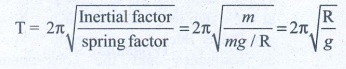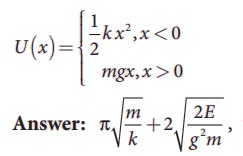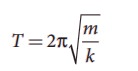Home | | Physics 11th std | Book Back Numerical Problems

# Book Back Numerical Problems

Physics : Oscillations : Book Back Exercise, Example Numerical Question with Answers, Solution : Book Back Numerical Problems

Oscillations (Physics)

Numerical Problems

1. Consider the Earth as a homogeneous sphere of radius R and a straight hole is bored in it through its centre. Show that a particle dropped into the hole will execute a simple harmonic motion such that its time period isSolution

Earth is assumed to be a homogeneous sphere.

Its centre is at O and Radius = R

The hole is bored straight through the centre along its diameter. The acceleration due to gravity at the surface of the earth = g

Mass of the body dropped inside the hole = m

After time t, the depth it reached (inside the earth) = d

The value of ‘g’ decreases with deportation.

So acceleration due to gravity at deportation = ‘g'

i.e.,g' = g(l -d//R) = g( (R-d) / R) ...(1)

Let y be the distance from the centre of the earth

Then y = Radius - distance = R - d

Substitute y in (1)

g' = g y/R

Now, force on the body of mass m due to this new acceleration g' will be

F = mg' = mgy /R

and this force is directed towards the mean position O.

The body dropped in the hole will execute S.H.M Spring factor k = mg/Radius2. Calculate the time period of the oscillation of a particle of mass m moving in the potential defined aswhere E is the total energy of the particle.

Solution

Length of simple pendulum l = 0.9 m

Inclined plane with the horizontal plane α = 45°

Time period of oscillation of simple pendulum T = ?3. Consider a simple pendulum of length = 0.9 m which is properly placed on a trolley rolling down on a inclined plane which is at θ = 45° with the horizontal. Assuming that the inclined plane is frictionless, calculate the time period of oscillation of the simple pendulum.

4. A piece of wood of mass m is floating erect in a liquid whose density is ρ. If it is slightly pressed down and released, then executes simple harmonic motion. Show that its time period of oscillation isSolution:

Spring factor of liquid = Aρg

Inertra factor of wood piece = m5. Consider two simple harmonic motion along x and y-axis having same frequencies but different amplitudes as = A sin (ω+ φ) (along x axis) and y = B sin ωt (along y axis). Then show thatand also discuss the special cases whenNote: when a particle is subjected to two simple harmonic motion at right angle to each other the particle may move along different paths. Such paths are called Lissajous figures.

a. y=B/A x equation  is  a  straight  line passing through origin with positive slope.

b.  y= - B/A x equation is a straight line passing through origin with negative slope.

c.equation is an ellipse whose center is origin.

d. x2+y2 = A2, equation is a circle whose center is origin .

e.equation is an ellipse (oblique ellipse which means tilted ellipse)

6. Show that for a particle executing simple harmonic motion

a. the average value of kinetic energy is equal to the average value of potential energy.

b. average potential energy = average kinetic energy = ½ (total energy)

Hint : average kinetic energy = <kinetic energy > = 1/T ∫0T(Kinetic energy) and average Potential energy = <Potential energy> =1/T ∫0T(Potential energy)7. Compute the time period for the following system if the block of mass m is slightly displaced vertically down from its equilibrium position and then released. Assume that the pulley is light and smooth, strings and springs are light.Case(a)

Pulley is fixed rigidly here. When the mass displace by y and the spring will also stretch by y. Therefore, F = T = kyCase(b)

Mass displace by y, pulley also displaces by y. T = 4ky.Tags : Oscillations | Physics , 11th Physics : UNIT 10 : Oscillations
Study Material, Lecturing Notes, Assignment, Reference, Wiki description explanation, brief detail
11th Physics : UNIT 10 : Oscillations : Book Back Numerical Problems | Oscillations | Physics